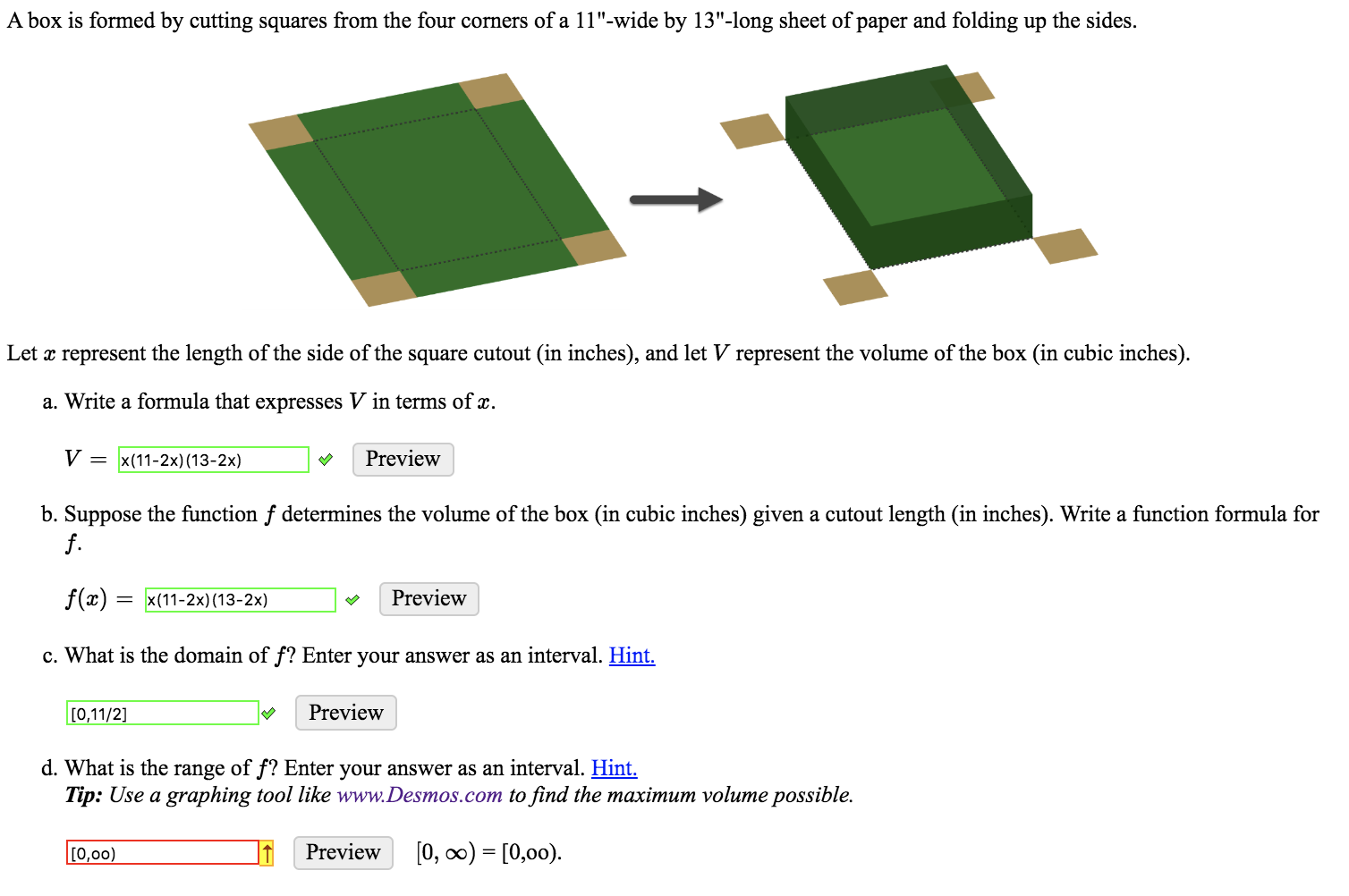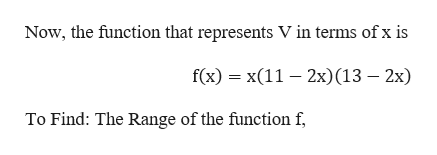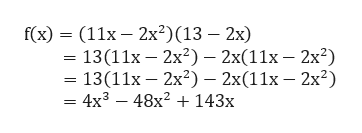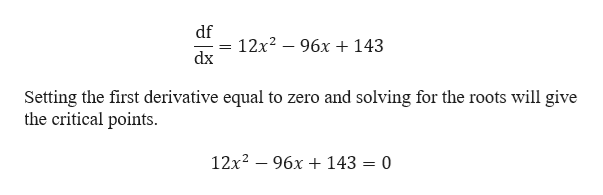# A box is formed by cutting squares from the four corners of a 11"-wide by 13"-long sheet of paper and folding up the sides.Let x represent the length of the side of the square cutout (in inches), and let V represent the volume of the box (in cubic inches).a. Write a formula that expresses V in terms of x.V = x(11-2x) (13-2x)Previewb. Suppose the function f determines the volume of the box (in cubic inches) given a cutout length (in inches). Write a function formula forf.f(x)Previewx(11-2x) (13-2x)c. What is the domain of f? Enter your answer as an interval. Hint.Preview[0,11/2]d. What is the range of f? Enter your answer as an interval. HintTip: Use a graphing tool like www.Desmos.com to find the maximum volume possible.[0, oo) [0,00)Preview[0,00)

Question
253 views

please respond to the box marked in redhelp_outlineImage TranscriptioncloseA box is formed by cutting squares from the four corners of a 11"-wide by 13"-long sheet of paper and folding up the sides. Let x represent the length of the side of the square cutout (in inches), and let V represent the volume of the box (in cubic inches). a. Write a formula that expresses V in terms of x. V = x(11-2x) (13-2x) Preview b. Suppose the function f determines the volume of the box (in cubic inches) given a cutout length (in inches). Write a function formula for f. f(x) Preview x(11-2x) (13-2x) c. What is the domain of f? Enter your answer as an interval. Hint. Preview [0,11/2] d. What is the range of f? Enter your answer as an interval. Hint Tip: Use a graphing tool like www.Desmos.com to find the maximum volume possible. [0, oo) [0,00) Preview [0,00) fullscreen
check_circle

star
star
star
star
star
1 Rating
Step 1

Given that a box is formed by cutting squares from the four corners. If x is the length of side cut out (in inches) and V represents the volume of the box.help_outlineImage TranscriptioncloseNow, the function that represents V in terms of x is f(x) — x(11 — 2х)(13 — 2х) To Find: The Range of the function f, fullscreen
Step 2

We find the range by finding the value of x such that the volume is maximum. Expanding the equation and simplifying gives,help_outlineImage Transcriptionclosef(х) — (11х — 2x?)(13 — 2х) - 13(11x - 2x2)- 2x(11x - 2x2 — 13(11х — 2x?) — 2х(11х — 2х?) 4x3 — 48х2 + 143х fullscreen
Step 3

Now let us take the first derivati...help_outlineImage Transcriptionclosedf 12x2 96x 143 dx Setting the first derivative equal to zero and solving for the roots will give the critical points. 12x2 96x 143 = 0 fullscreen

### Want to see the full answer?

See Solution

#### Want to see this answer and more?

Solutions are written by subject experts who are available 24/7. Questions are typically answered within 1 hour.*

See Solution
*Response times may vary by subject and question.
Tagged in

### Equations and In-equations